Courses

# Divergence, Curl and Gradient Physics Notes | EduRev

## Physics : Divergence, Curl and Gradient Physics Notes | EduRev

The document Divergence, Curl and Gradient Physics Notes | EduRev is a part of the Physics Course Basic Physics for IIT JAM.
All you need of Physics at this link: Physics

Vector and Scalar Fields

Definition

Let C : R→F                     C        :                              R                                3                          →        F              {\displaystyle C:\mathbb {R} ^{3}\to F}  , where F                    F              {\displaystyle F}    is a field. We say that C                    C              {\displaystyle C}    is a scalar field

In the physical world, examples of scalar fields are

(i) The electrostatic potential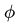ϕ              {\displaystyle \phi }    in space

(ii) The distribution of temperature in a solid body, T(r)            T        (                  r                )              {\displaystyle T(\mathbf {r} )}

Definition

Let V                    V              {\displaystyle V}    be a vector space. Let F : R3 → V                              F                :                              R                                3                          →        V              {\displaystyle \mathbf {F} :\mathbb {R} ^{3}\to V}   , we say that F                              F                      {\displaystyle \mathbf {F} }    is a vector field; it associates a vector from V                    V              {\displaystyle V}    with every point of R3                                          R                                3                                {\displaystyle \mathbb {R} ^{3}}   .

In the physical world, examples of vector fields are

(i) The electric and magnetic fields in space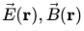E              →                                      (                  r                )        ,                                            B              →                                      (                  r                )              {\displaystyle {\vec {E}}(\mathbf {r} ),{\vec {B}}(\mathbf {r} )}

(ii) The velocity field in a fluid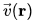Let C                    C              {\displaystyle C}    be a scalar field. We define the gradient as an "operator"                     ∇              {\displaystyle \nabla }   ∇ mapping the field C                   C              {\displaystyle C}    to a vector in R3                                          R                                3                                {\displaystyle \mathbb {R} ^{3}}    such that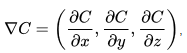or as is commonly denoted                     ∇        C        =                                            ∂              C                                      ∂              x                                                                          x              ^                                      +                                            ∂              C                                      ∂              y                                                                          y              ^                                      +                                            ∂              C                                      ∂              z                                                                          z              ^                                            {\displaystyle \nabla C={\frac {\partial C}{\partial x}}{\hat {x}}+{\frac {\partial C}{\partial y}}{\hat {y}}+{\frac {\partial C}{\partial z}}{\hat {z}}}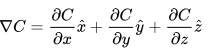We shall encounter the physicist's notion of "operator" before defining it formally in the chapter Hilbert Spaces. It can be loosely thought of as "a function of functions"

Recall from multivariable calculus that the total derivative of a function f : R3 →R                     f        :                              R                                3                          →                  R                      {\displaystyle f:\mathbb {R} ^{3}\to \mathbb {R} }   at a ∈ R                              a                ∈                              R                                3                                {\displaystyle \mathbf {a} \in \mathbb {R} ^{3}}   is defined as the linear transformation A                    A              {\displaystyle A}    that satisfies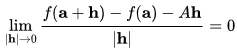In the usual basis, we can express as the row matrix                               f          ′                (                  a                )        =        A        =                                            (                                                                                                                                                                        ∂                            f                                                                                ∂                            x                                                                                                                                                                                                                                                        ∂                            f                                                                                ∂                            y                                                                                                                                                                                                                                                        ∂                            f                                                                                ∂                            z                                                                                                                                                          )                                            {\displaystyle f'(\mathbf {a} )=A=\displaystyle {\begin{pmatrix}{\tfrac {\partial f}{\partial x}}&{\tfrac {\partial f}{\partial y}}&{\tfrac {\partial f}{\partial z}}\\\end{pmatrix}}}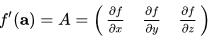It is customary to denote vectors as column matrices. Thus we may write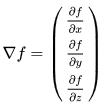The transpose of a matrix given by constituents aij                              a                      i            j                                {\displaystyle a_{ij}}    is the matrix with constituents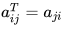Thus, the gradient is the transpose of the total derivative.

Divergence

Let F : R3 → R3                              F                :                              R                                3                          →                              R                                3                                {\displaystyle \mathbf {F} :\mathbb {R} ^{3}\to \mathbb {R} ^{3}}    be a vector field and let F                              F                      {\displaystyle \mathbf {F} }    be differentiable.

We define the divergence as the operator (∇.)                    (        ∇        ⋅        )              {\displaystyle (\nabla \cdot )}    mapping F                              F                      {\displaystyle \mathbf {F} }    to a scalar such that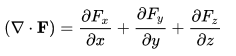Curl

Let F : R3 → R3                              F                :                              R                                3                          →                              R                                3                                {\displaystyle \mathbf {F} :\mathbb {R} ^{3}\to \mathbb {R} ^{3}}    be a vector field and let F                              F                      {\displaystyle \mathbf {F} }    be differentiable.

We define the curl as the operator ( ∇ x)                    (        ∇        ×        )              {\displaystyle (\nabla \times )}    mapping F                              F                      {\displaystyle \mathbf {F} }    to a linear transformation from R3                                          R                                3                                {\displaystyle \mathbb {R} ^{3}}    onto itself such that the linear transformation can be expressed as the matrix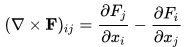written in short as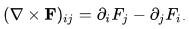Here, x1,x2,x                              x                      1                          ,                  x                      2                          ,                  x                      3                                {\displaystyle x_{1},x_{2},x_{3}}   denote                     x        ,        y        ,        z              {\displaystyle x,y,z}   x,y,z and so on.

the curl can be explicitly given by the matrix:                     ∇        ×                  F                =                              (                                                            0                                                                      ∂                                          1                                                                            F                                          2                                                        −                                      ∂                                          2                                                                            F                                          1                                                                                                            ∂                                          1                                                                            F                                          3                                                        −                                      ∂                                          3                                                                            F                                          1                                                                                                                                        ∂                                          2                                                                            F                                          1                                                        −                                      ∂                                          1                                                                            F                                          2                                                                                        0                                                                      ∂                                          2                                                                            F                                          3                                                        −                                      ∂                                          3                                                                            F                                          2                                                                                                                                        ∂                                          2                                                                            F                                          1                                                        −                                      ∂                                          1                                                                            F                                          3                                                                                                            ∂                                          3                                                                            F                                          2                                                        −                                      ∂                                          2                                                                            F                                          3                                                                                        0                                                      )                                {\displaystyle \nabla \times \mathbf {F} ={\begin{pmatrix}0&\partial _{1}F_{2}-\partial _{2}F_{1}&\partial _{1}F_{3}-\partial _{3}F_{1}\\\partial _{2}F_{1}-\partial _{1}F_{2}&0&\partial _{2}F_{3}-\partial _{3}F_{2}\\\partial _{2}F_{1}-\partial _{1}F_{3}&\partial _{3}F_{2}-\partial _{2}F_{3}&0\\\end{pmatrix}}}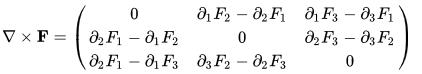v              →                                      (                  r                )              {\displaystyle {\vec {v}}(\mathbf {r} )}

this notation is also sometimes used to denote the vector exterior or cross product,                     ∇        ×                  F                =        (                  ∂                      2                                    F                      3                          −                  ∂                      3                                    F                      2                          )                                            x              ^                                      +        (                  ∂                      1                                    F                      3                          −                  ∂                      3                                    F                      1                          )                                            y              ^                                      +        (                  ∂                      1                                    F                      2                          −                  ∂                      2                                    F                      1                          )                                            z              ^                                            {\displaystyle \nabla \times \mathbf {F} =(\partial _{2}F_{3}-\partial _{3}F_{2}){\hat {x}}+(\partial _{1}F_{3}-\partial _{3}F_{1}){\hat {y}}+(\partial _{1}F_{2}-\partial _{2}F_{1}){\hat {z}}}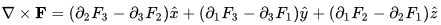Offer running on EduRev: Apply code STAYHOME200 to get INR 200 off on our premium plan EduRev Infinity!

,

,

,

,

,

,

,

,

,

,

,

,

,

,

,

,

,

,

,

,

,

,

,

,

;